Courses

# NCERT Solutions - Polynomials, Class 10, Maths Class 10 Notes | EduRev

## Class 10 : NCERT Solutions - Polynomials, Class 10, Maths Class 10 Notes | EduRev

The document NCERT Solutions - Polynomials, Class 10, Maths Class 10 Notes | EduRev is a part of Class 10 category.
All you need of Class 10 at this link: Class 10

Page No: 28

Exercises 2.1

1. The graphs of y = p(x) are given in following figure, for some polynomials p(x). Find the number of zeroes of p(x), in each case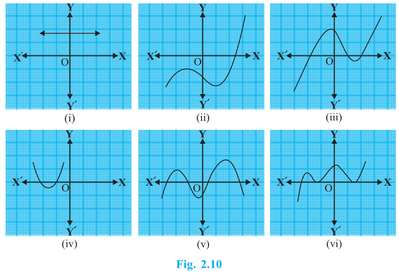(i) The number of zeroes is 0 as the graph does not cut the x-axis at any point.
(ii) The number of zeroes is 1 as the graph intersects the x-axis at only 1 point.
(iii) The number of zeroes is 3 as the graph intersects the x-axis at 3 points.
(iv) The number of zeroes is 2 as the graph intersects the x-axis at 2 points.
(v) The number of zeroes is 4 as the graph intersects the x-axis at 4 points.
(vi) The number of zeroes is 3 as the graph intersects the x-axis at 3 points.

Page No: 33

Exercise 2.2

1. Find the zeroes of the following quadratic polynomials and verify the relationship between the zeroes and the coefficients.
(i) x2 – 2x – 8
(ii) 4s2 – 4s 1
(iii) 6x2 – 3 – 7x
(iv) 4u2 8u
(v) t2 – 15
(vi) 3x2 x – 4

(i) x2 – 2x – 8
= (x - 4) (x  2)
The value of x2 – 2x – 8 is zero when x - 4 = 0 or x 2 = 0, i.e., when x = 4 or x = -2
Therefore, the zeroes of x2 – 2x – 8 are 4 and -2.

Sum of zeroes = 4 (-2) = 2 = -(-2)/1 = -(Coefficient of x)/Coefficient of x2

Product of zeroes = 4 × (-2) = -8 = -8/1 = Constant term/Coefficient of x2

(ii) 4s2 – 4s + 1= (2s-1)2
The value of 4s2 - 4s +1 is zero when 2s - 1 = 0, i.e., s = 1/2

Therefore, the zeroes of 4s2 - 4s + 1 are 1/2 and 1/2.

Sum of zeroes = 1/2 1/2 = 1 = -(-4)/4 = -(Coefficient of s)/Coefficient of s2
Product of zeroes = 1/2 × 1/2 = 1/4 = Constant term/Coefficient of s2.

(iii) 6x2 – 3 – 7x
6x– 7– 3
= (3x  1) (2x - 3)
The value of 6x2 - 3 - 7x is zero when 3x + 1 = 0 or 2x - 3 = 0, i.e., x = -1/3 or x = 3/2

Therefore, the zeroes of 6x2 - 3 - 7x are -1/3 and 3/2.

Sum of zeroes = -1/3 3/2 = 7/6 = -(-7)/6 = -(Coefficient of x)/Coefficient of x2
Product of zeroes = -1/3 × 3/2 = -1/2 = -3/6 = Constant term/Coefficient of x2.

(iv) 4u2   8u
4u2  8u  0
= 4u(u 2)
The value of 4u2 8u is zero when 4u = 0 or u 2 = 0, i.e., u = 0 or u = - 2
Therefore, the zeroes of 4u2 8u are 0 and - 2.
Sum of zeroes = 0 (-2) = -2 = -(8)/4 = -(Coefficient of u)/Coefficient of u2
Product of zeroes = 0 × (-2) = 0 = 0/4 = Constant term/Coefficient of u2.

(v) t2 – 15
t- 0.t - 15
= (- √15) (t  √15)
The value of t2 - 15 is zero when t - √15 = 0 or t  √15 = 0, i.e., when t = √15 or = -√15
Therefore, the zeroes of t2 - 15 are √15 and -√15.Sum of zeroes = √15   -√15 = 0 = -0/1 = -(Coefficient of t)/Coefficient of t2
Product of zeroes = (√15) (-√15) = -15 = -15/1 = Constant term/Coefficient of u2.

(vi) 3x2 x – 4
= (3x - 4) (x  1)
The value of 3x2 x – 4 is zero when 3x - 4 = 0 and x  1 = 0,i.e., when x = 4/3 or x = -1
Therefore, the zeroes of 3x2 x – 4 are 4/3 and -1.
Sum of zeroes = 4/3  (-1) = 1/3 = -(-1)/3 = -(Coefficient of x)/Coefficient of x2
Product of zeroes = 4/3 × (-1) = -4/3 = Constant term/Coefficient of x2.

2. Find a quadratic polynomial each with the given numbers as the sum and product of its zeroes respectively.

(i) 1/4 , -1

(ii) √2 , 1/3

(iii) 0, √5

(iv) 1,1

(v) -1/4 ,1/4

(vi) 4,1

(i) 1/4 , -1
Let the polynomial be ax2  bx  c, and its zeroes be α and ß
α  ß = 1/4 = -b/a
αß = -1 = -4/4 = c/a
If a = 4, then b = -1, c = -4
Therefore, the quadratic polynomial is 4x2 - x -4.

(ii) √2 , 1/3
Let the polynomial be ax2   bx   c, and its zeroes be α and ß
α  ß = √2 = 3√2/3 = -b/a
αß = 1/3 = c/a
If a = 3, then b = -3√2, c = 1
Therefore, the quadratic polynomial is 3x2 -3√2x  1.

(iii) 0, √5
Let the polynomial be ax2   bx   c, and its zeroes be α and ß
α  ß = 0 = 0/1 = -b/a
αß = √5 = √5/1 = c/a
If a = 1, then b = 0, c = √5
Therefore, the quadratic polynomial is x2  √5.

(iv) 1, 1
Let the polynomial be ax2   bx   c, and its zeroes be α and ß
α  ß = 1 = 1/1 = -b/a
αß = 1 = 1/1 = c/a
If a = 1, then b = -1, c = 1
Therefore, the quadratic polynomial is x2x  1.

(v) -1/4 ,1/4
Let the polynomial be ax2   bx   c, and its zeroes be α and ß
α  ß = -1/4 = -b/a
αß = 1/4 = c/a
If a = 4, then b = 1, c = 1
Therefore, the quadratic polynomial is 4x2   x  1.

(vi) 4,1
Let the polynomial be ax2   bx   c, and its zeroes be α and ß
α  ß = 4 = 4/1 = -b/a
αß = 1 = 1/1 = c/a
If a = 1, then b = -4, c = 1
Therefore, the quadratic polynomial is x2 - 4x  1.

Page No: 36

Exercise 2.3

1. Divide the polynomial p(x) by the polynomial g(x) and find the quotient and remainder in each of the following:

(i) p(x) = x3 – 3x2  5x – 3, g(x) = x2 – 2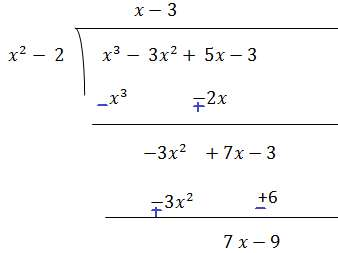Quotient = x-3 and remainder 7x - 9

(ii) p(x) = x4 – 3x2  4x 5, g(x) = x2  1 – x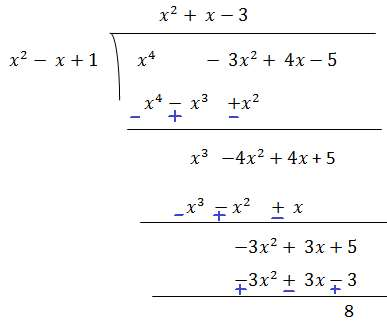Quotient = x2  - 3 and remainder 8

(iii) p(x) = x4 – 5x  6, g(x) = 2 – x2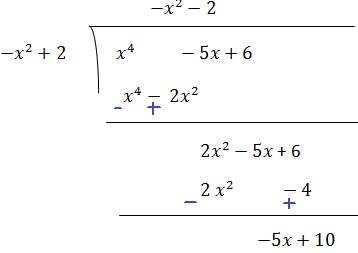Quotient = -x2 -2 and remainder -5x 10

2. Check whether the first polynomial is a factor of the second polynomial by dividing the second polynomial by the first polynomial:

(i) t2 – 3,  2t4 3t3 – 2t2 – 9t – 12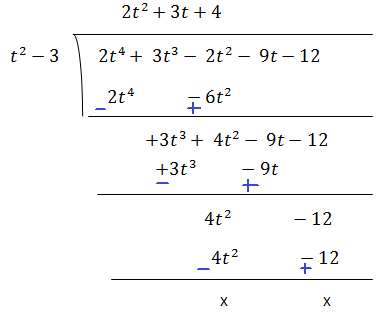t2 – 3 exactly divides  2t4  3t3 – 2t2 – 9t – 12 leaving no remainder. Hence, it is a factor of  2t4  3t3 – 2t2 – 9t – 12.

(ii) x2 3x 1, 3x4 5x3 – 7x2 2x 2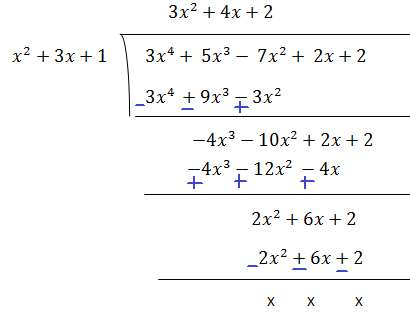x2  3x  1 exactly divides 3x4  5x3 – 7x2  2x  2 leaving no remainder. Hence, it is factor of 3x4  5x3 – 7x2  2x  2.

(iii) x3 – 3x 1, x5 – 4x3 x2 3x 1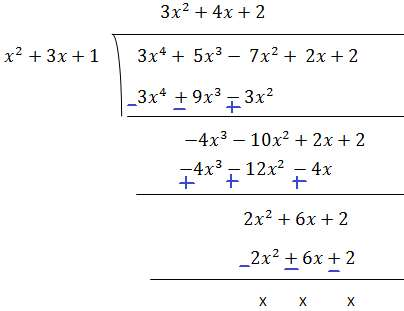x2  3x  1 exactly divides 3x4  5x3 – 7x2  2x  2 leaving no remainder. Hence, it is factor of 3x4  5x3 – 7x2  2x  2.

(iii) x3 – 3x 1, x5 – 4x3 x2 3x 1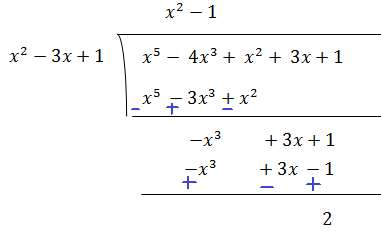x3 – 3x  1 didn't divides exactly x5 – 4x3   x2  3x  1 and leaves 2 as remainder. Hence, it not a factor of x5 – 4x3   x2  3x  1.

3. Obtain all other zeroes of 3x4 6x3 – 2x2 – 10x – 5, if two of its zeroes are √(5/3) and - √(5/3).

p(x) = 3x4  6x3 – 2x2 – 10x – 5
Since the two zeroes are √(5/3) and - √(5/3).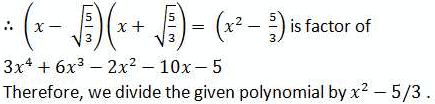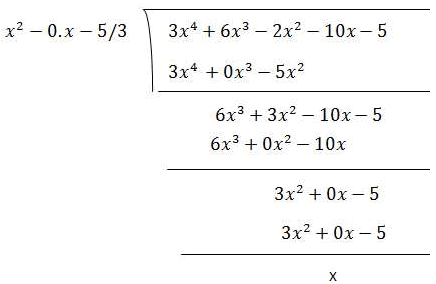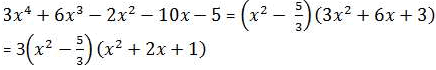We factorize x2 + 2x + 1

= ( + 1)2

Therefore, its zero is given by x  + 1 = 0

x = -1

As it has the term (x + 1)2 , therefore, there will be 2 zeroes at x = - 1.
Hence, the zeroes of the given polynomial are √(5/3) and - √(5/3), - 1 and - 1.

4.  On dividing x3 - 3x2  x 2 by a polynomial g(x), the quotient and remainder were x - 2 and  -2x  4, respectively. Find g(x).

Here in the given question,

Dividend = x3 - 3x2  x 2

Quotient = x - 2

Remainder = -2x  4

Divisor = g(x)

We know that,

Dividend = Quotient × Divisor  Remainder

⇒ x3 - 3x2  x 2 = (x - 2) × g(x)  (-2x  4)⇒ x3 - 3x2  x 2 - (-2x  4) = (x - 2) × g(x)
⇒ x3 - 3x2  3x - 2 = (x - 2) × g(x)
g(x) =  (x3 - 3x2  3x - 2)/(x - 2)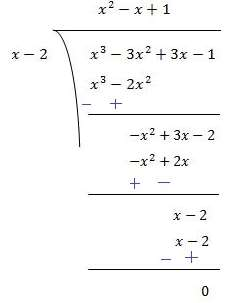∴ g(x) = (x2 - x + 1)

5.Give examples of polynomial p(x), g(x), q(x) and r(x), which satisfy the division algorithm and
(i) deg p(x) = deg q(x)
(ii) deg q(x) = deg r(x)
(iii) deg r(x) = 0

(i) Let us assume the division of 6x2 2x 2 by 2
Here, p(x) = 6x2 2x 2
g(x) = 2
q(x) = 3x2 x 1
r(x) = 0
Degree of p(x) and q(x) is same i.e. 2.
Checking for division algorithm,
p(x) = g(x) × q(x) r(x)
Or, 6x2 2x 2 = 2x (3x2 1)
Hence, division algorithm is satisfied.

(ii) Let us assume the division of x3 x by x2,
Here, p(x) = x3 x
g(x) = x2
q(x) = x and r(x) = x
Clearly, the degree of q(x) and r(x) is the same i.e., 1.
Checking for division algorithm,
p(x) = g(x) × q(x) r(x)
x3 x = (x2 ) × x x
x3 x = x3 x
Thus, the division algorithm is satisfied.

(iii) Let us assume the division of x3 1 by x2.
Here, p(x) = x3 1
g(x) = x2
q(x) = x and r(x) = 1
Clearly, the degree of r(x) is 0.
Checking for division algorithm,
p(x) = g(x) × q(x) r(x)
x3  1 = (x2 ) × 1
x3 1 = x3 1
Thus, the division algorithm is satisfied.

Offer running on EduRev: Apply code STAYHOME200 to get INR 200 off on our premium plan EduRev Infinity!

,

,

,

,

,

,

,

,

,

,

,

,

,

,

,

,

,

,

,

,

,

,

,

,

,

,

,

;